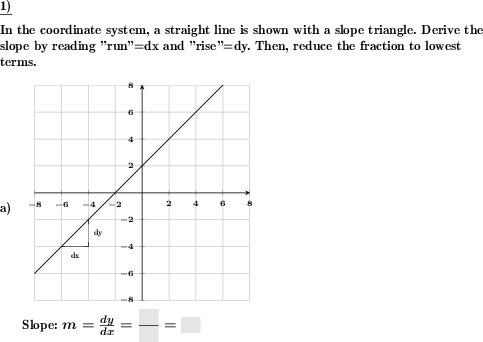Custom math worksheets at your fingertips# Details for problem "Slope to be derived from given slope triangle"

Quickname: 2201

Elementary School, Primary School, Junior High School, Middle School, High School.

## Summary

The slope shall be derived from a straight line with a gradient triangle in the coordinate system.

## Example## Description

For a given straight line, the slope is to be determined by reading dx and dy from a given slope triangle. The straight line is provided with a slope triangle in a coordinate system with the problem statement. The triangle is labeled "dx" for the run and "dy" for the rise. The task requires that the values read off be properly written in a fraction, reduced to lowest terms if necessary and thus the slope determined.

The intersection of the line and the Y-axis can be chosen to lie on only full or also half coordinate plane squares (vertical divisions).

With respect to the coordinate system, it can be set whether only quadrant one is to be covered with positive X and Y coordinates, or also the quadrants containing coordinates with negative signs. The coordinate system can be plotted in three different sizes.

The slope is chosen randomly, but can also be restricted to be positive or negative only

Download free printable worksheets for this math problem here. The worksheet contains the problems only, the solution sheet includes the answers. Just click on the respective link.

•Worksheet 1Solution sheet with answers
•Worksheet 2Solution sheet with answers
•Worksheet 3Solution sheet with answers

If you can not see the solution sheets for download, they may be filtered out by an ad blocker that you may have installed. If this is the case, please allow ads for this page and reload the page. The solution sheets will then reappear.

• Do these sample worksheets do not really fit?
• Do you need more math worksheets, with a different level of difficulty?
• Would you like to combine different problems on a worksheet and adjust them to your needs?
• As a teacher, you can put together your own worksheets using the automatically generated math problems provided.
With a free initial credit, you can start creating your own math worksheets in a few minutes.

You can try it for free! Register here, to create custom worksheets now!

## Customization options for this problem

Parameter
Possible values
Number of problems
1, 2
Yes, No
Slope
pos & neg, positive, negative
Sub-square rises
Yes, No
Size
small, medium, large

## Similar problems

Remark
Description
Draw the slope triangle, too
In a coordinate system with a given straight line a slope triangle is to be drawn.